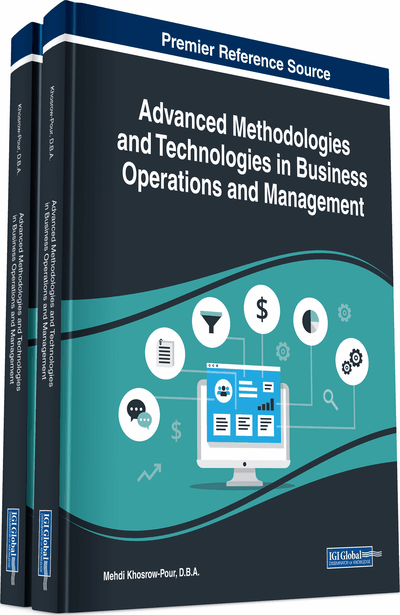# Comprehensible Explanation of Predictive Models

Marko Robnik-Šikonja (University of Ljubljana, Slovenia)
DOI: 10.4018/978-1-5225-7362-3.ch046

## Abstract

The most successful prediction models (e.g., SVM, neural networks, or boosting) unfortunately do not provide explanations of their predictions. In many important applications of machine learning, the comprehension of the decision process is of utmost importance and dominates the classification accuracy (e.g., in business and medicine). This chapter introduces general explanation methods that are independent of the prediction model and can be used with all classification models that output probabilities. It explains how the methods work and graphically explains models' decisions for new unlabeled cases. The approach is put in the context of applications from medicine, business, and macro-economy.
Chapter Preview
Top

## Background

In a typical data science problem setting, users are concerned with both prediction accuracy and the interpretability of the prediction model. Complex models have potentially higher accuracy but are more difficult to interpret. This can be alleviated either by sacrificing some prediction accuracy for a more transparent model or by using an explanation method that improves the interpretability of the model. Explaining predictions is straightforward for symbolic models such as decision trees, decision rules, and inductive logic programming, where the models give an overall transparent knowledge in a symbolic form. Therefore, to obtain the explanations of predictions, one simply has to read the rules in the corresponding model. Whether such an explanation is comprehensive in the case of large trees and rule sets is questionable.

## Key Terms in this Chapter

Quality of the Explanation: Can be judged by several criteria: accuracy (generalization ability), fidelity (how well the explanation reflects behavior of the model), consistency (similarity of behavior for different models trained on the same task), and comprehensibility (readability and size of the extracted knowledge).

Domain Level Explanation: Tries to find the true causal relationship between the dependent and independent variables. Typically this level is unreachable except for artificial domains where all the relations as well as the probability distributions are known in advance.

Instance Level Explanation: Explanation of prediction of a single instance with a given model, that is, at the model level.

Feature Contribution: A value assigned to a feature (or its value) that is proportional to the feature’s share in the model’s prediction for an instance.

Expressive Power of Explanation: Describes the language of extracted knowledge: propositional logic (that is, if-then rules), nonconventional logic (for example, fuzzy logic), first-order logic, and finite state machines (deterministic, nondeterministic, stochastic).

Model Level Explanation: Aims is to make transparent the prediction process of a particular model. Empirical observations show that better models (with higher prediction accuracy) enable better explanation at the domain level.

Marginal Effect of a Feature: A difference between model’s prediction with the feature and without it, holding all other features intact.

Portability of Explanation: Describes how well the explanation technique covers the set of available models.

Attribute Evaluation: A data mining procedure which estimates the utility of attributes for given task (usually prediction). Attribute evaluation is used in many data mining tasks, for example in feature subset selection, feature weighting, feature ranking, feature construction, decision and regression tree building, data discretization, visualization, and comprehension.

## Complete Chapter List

Search this Book:
Reset